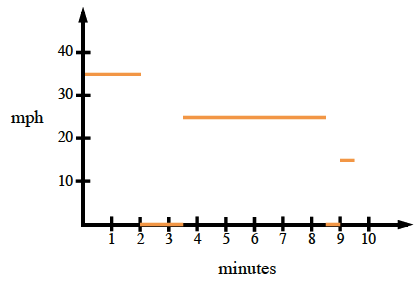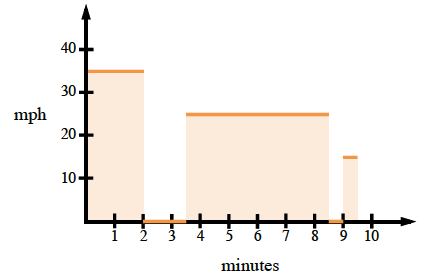### Home > PC > Chapter 9 > Lesson 9.3.3 > Problem9-127

9-127.

Sean drove $35$ mph for $2$ minutes, sat at a stop light for $1.5$ minutes, drove $25$ mph through a construction zone for $5$ minutes, stopped for a pedestrian for $0.5$ minutes, and then continued home at $15$ mph for $0.5$ minutes.

1. Make a velocity graph of their trip.2. Calculate the total distance traveled.

$(35)\left(\frac{1}{30} \right )+... \text{Why }\frac{1}{30}?$